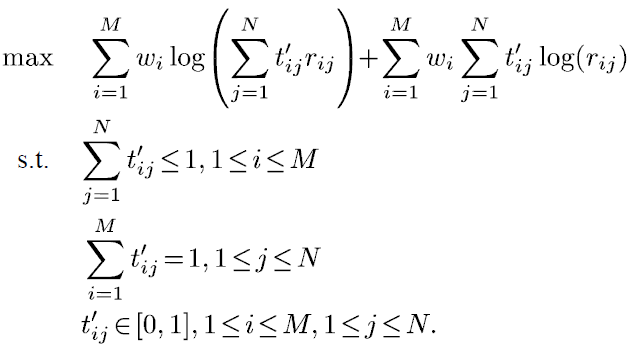# Solving an NLP using 2-dimension variables

I intend to solve the following formulation using 2-dimension variables.the following lines shows my code in cvx and it seems works fine without any error and return the results successfully.

``````% variable w_{i} is ignored (w_{i} = 1)
r =  [ 54    36; 36    36;  24    36 ];
m = 3;
n = 2;
cvx_begin
variable tp(m,n);
f1 = sum(log(sum(tp(1:m,1:n).*r(1:m,1:n))));
g1 = sum(sum(tp(1:m,1:n).*log(r(1:m,1:n))));
maximize (f1 + g1)
subject to
0 <= tp <= 1;
sum(tp(2)) <= 1;
sum(tp(1)) == 1;
cvx_end
``````

The returned results:

``````Successive approximation method to be employed.
For improved efficiency, SDPT3 is solving the dual problem.
SDPT3 will be called several times to refine the solution.
Original size: 22 variables, 7 equality constraints
2 exponentials add 16 variables, 10 equality constraints
-----------------------------------------------------------------
Cones  |             Errors              |
Mov/Act | Centering  Exp cone   Poly cone | Status
--------+---------------------------------+---------
2/  2 | 2.963e+00  9.612e-01  0.000e+00 | Solved
2/  2 | 7.430e-01  4.706e-02  1.279e-08 | Solved
2/  2 | 3.057e-02  7.469e-05  2.723e-08 | Solved
0/  2 | 3.035e-04  5.209e-08  4.473e-08 | Solved
-----------------------------------------------------------------
Status: Solved
Optimal value (cvx_optval): +30.9194

>> tp

tp = 1.0000 1.0000
1.0000 1.0000
1.0000 1.0000
1.0000 1.0000
``````

although it seems cvx has solved the problem, when I check the calculated tp values w.r.t the defined constraints, they have been violated! for instance, the sum of each row of tp(i,j) has to be less equal than 1, but it doesn’t follow this constraint for all the rows. the same concern exists for the second constraint.

Is there anything that I missed in my coding part?

Your sum constraints, as implemented in your CXV code, are not what you intended. Each of your sum constraints as implemented is constraining (the sum of) a single entry of the vec’d version of the tp matrix. I believe this is what you want for the sum constraints:

``````sum(tp,2) <= 1
sum(tp,1) == 1
``````

I will leave it to you to determine whether your code for f1 and g1 is correct. Using CVX2.1, I got

``````Error using subsindex
Function 'subsindex' is not defined for values of class 'cvx'.
``````

when I tried to run your code.

thanks mark. it works now! I updated my code regarding your point as follows:

``````r =  [ 54    36;   36    36;  24    36 ];
m = 3;
n = 2;
cvx_begin
variable tp(m,n);
f1 = sum(log(sum(tp(1:m,1:n).*r(1:m,1:n))));
g1 = sum(sum(tp(1:m,1:n).*log(r(1:m,1:n))));
maximize (f1 + g1)
subject to
0 <= tp <= 1;
sum(tp,2) <= 1;
sum(tp,1) == 1;
cvx_end
``````

I can run the code without error in matlab and the results are:

``````Successive approximation method to be employed.
For improved efficiency, SDPT3 is solving the dual problem.
SDPT3 will be called several times to refine the solution.
Original size: 23 variables, 8 equality constraints
2 exponentials add 16 variables, 10 equality constraints
-----------------------------------------------------------------
Cones  |             Errors              |
Mov/Act | Centering  Exp cone   Poly cone | Status
--------+---------------------------------+---------
2/  2 | 2.518e+00  6.357e-01  0.000e+00 | Solved
2/  2 | 4.658e-01  1.773e-02  0.000e+00 | Solved
2/  2 | 5.539e-03  2.405e-06  0.000e+00 | Solved
0/  0 | 1.014e-04  0.000e+00  0.000e+00 | Solved
-----------------------------------------------------------------
Status: Solved
Optimal value (cvx_optval): +15.145

>> tp

tp =

1.0000    0.0000
0.0000    0.4980
0.0000    0.5020
``````

now the constraints are not violated and I got the answer as well. thanks again. it was a big help.